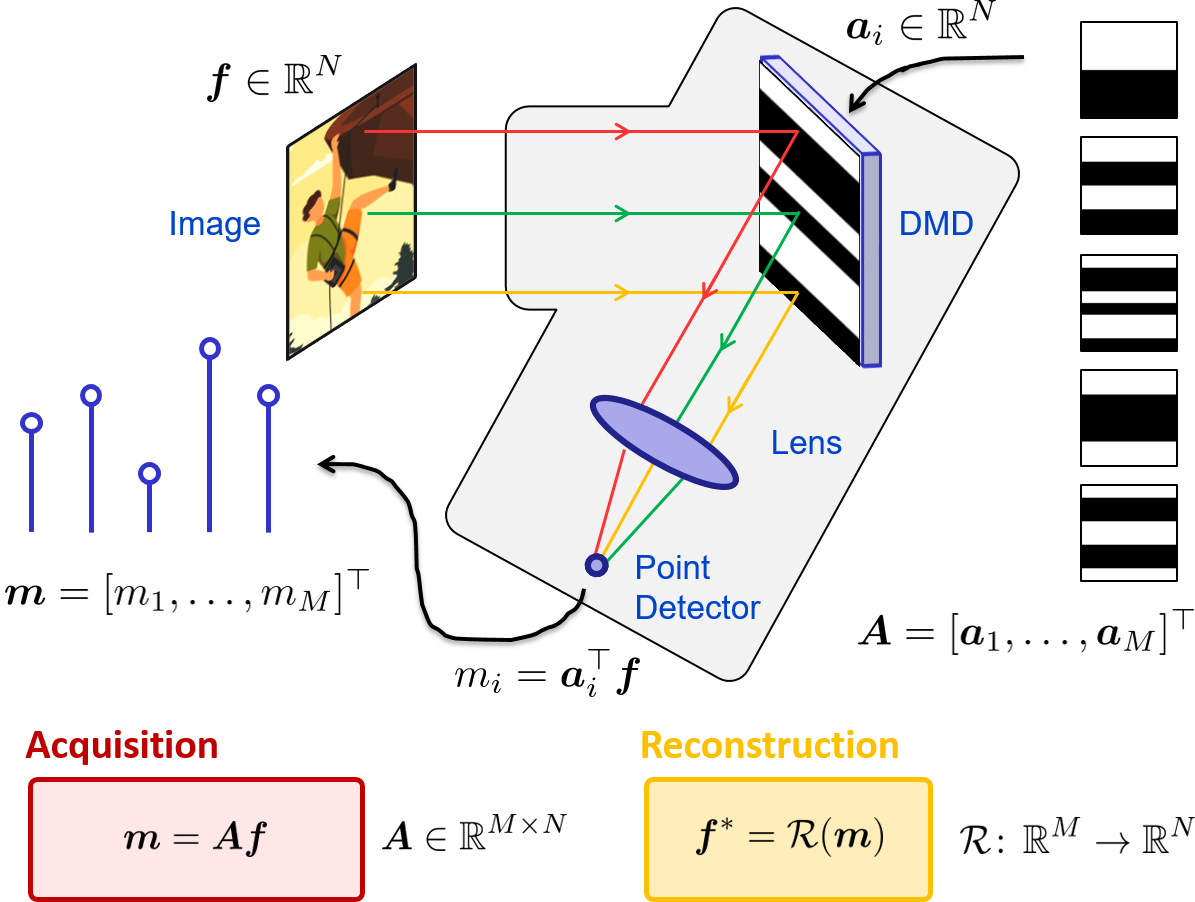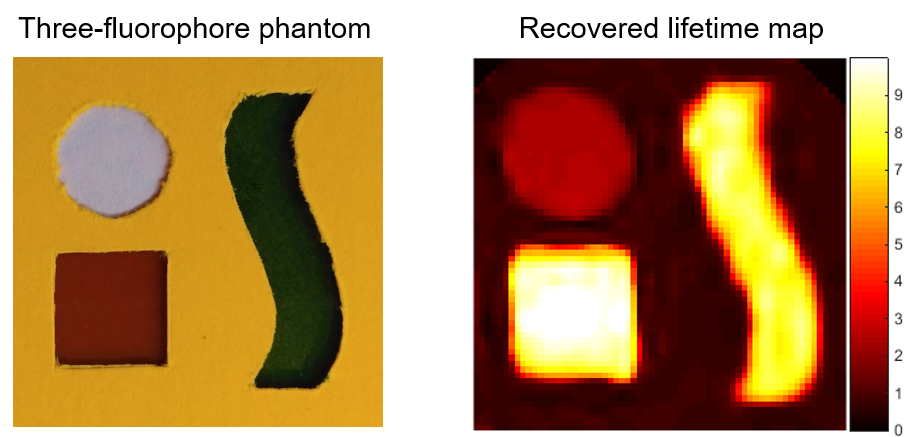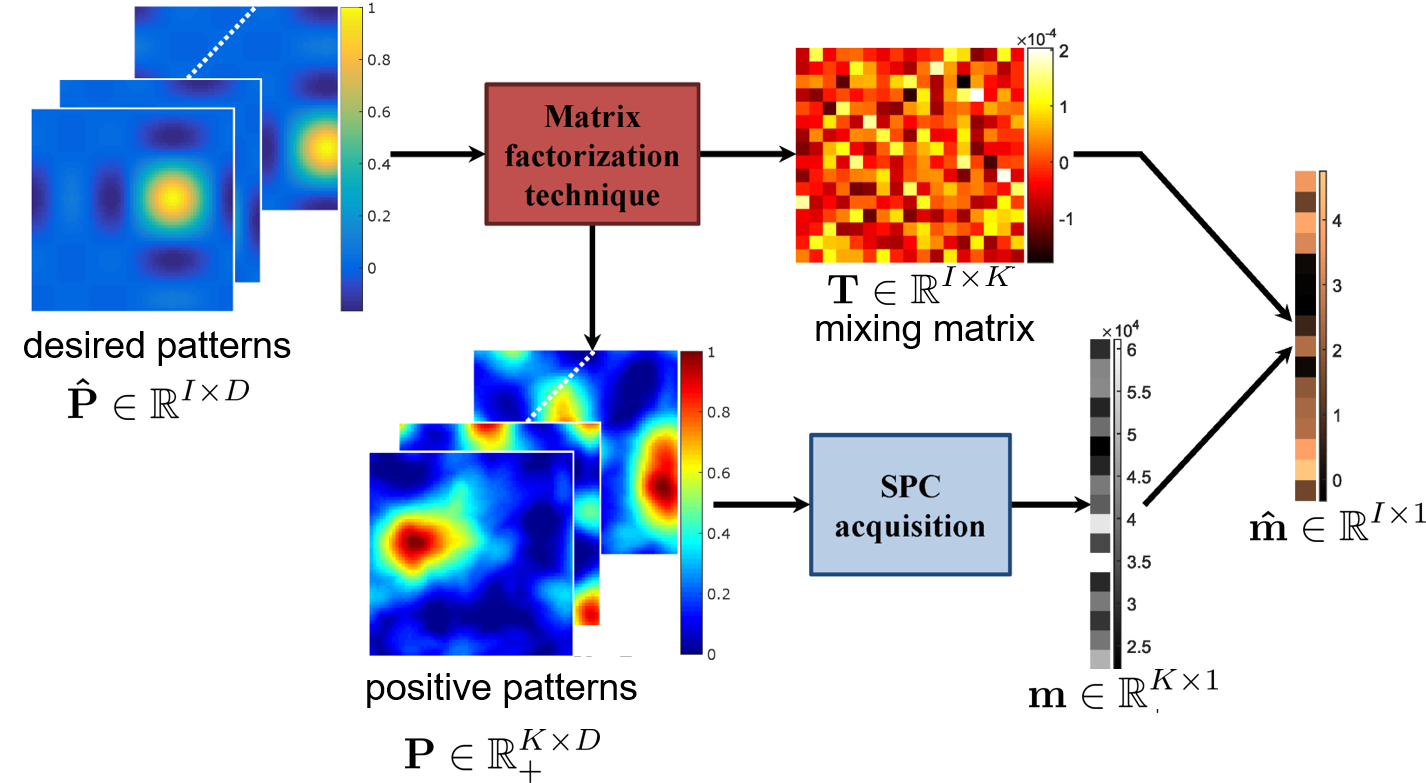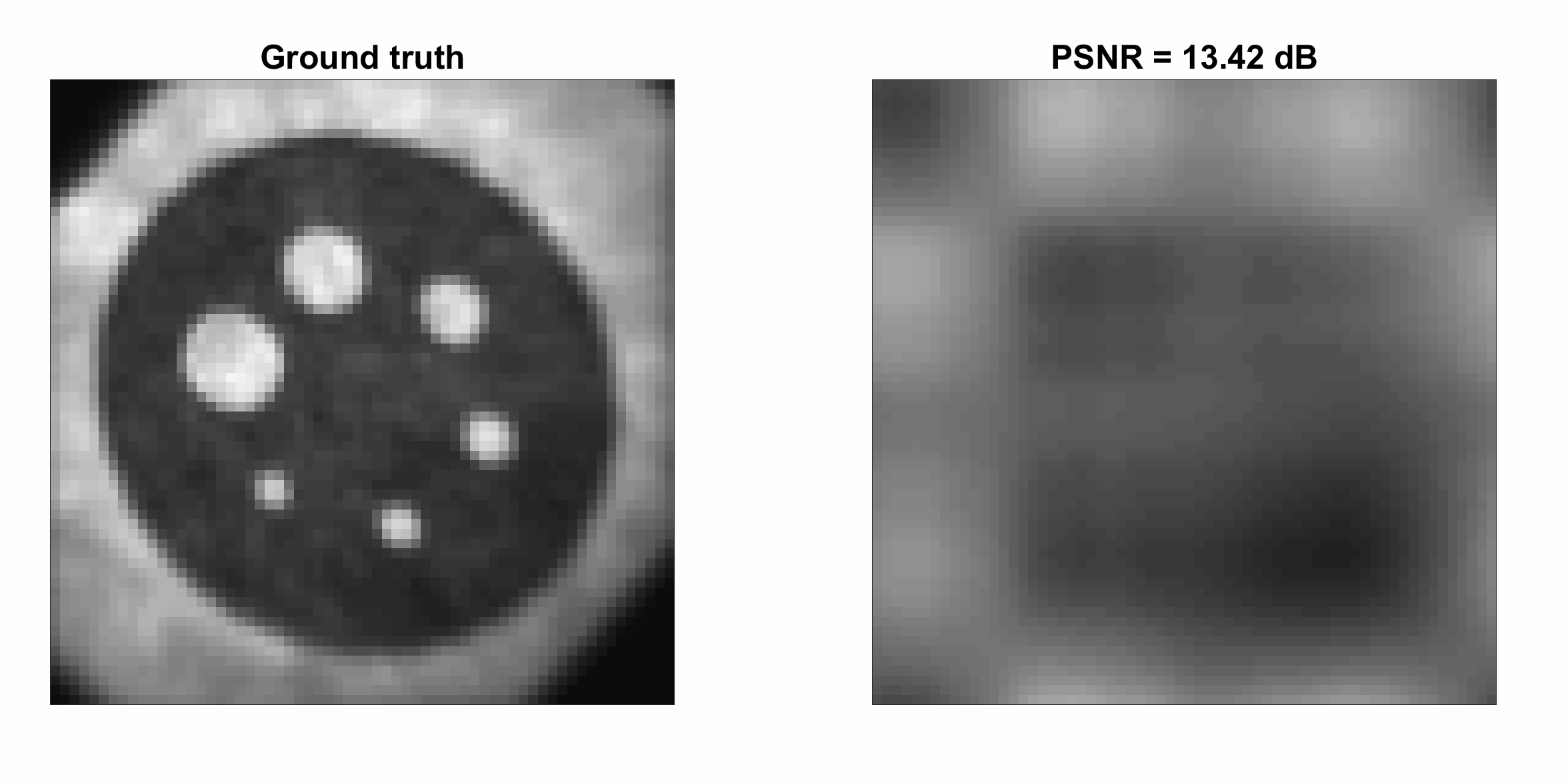## SINGLE-PIXEL IMAGING

### Overview

Single-pixel imaging is an emerging paradigm that allows high-quality images to be provided by a device that is only equipped with a single point detector. Such a device is referred to as a single-pixel camera. A common implementation of the single-pixel camera relies on the use of a digital micromirror device, which is a spatial light modulator (see Figure below).A single-pixel camera is an experimental set-up that can be used to measure the inner product of a scene under view - the image - with any user-defined pattern. Let $\mathbf{f} \in \mathbb{R}^{N^2 \times 1}$ be the $N \times N$ image of the object and $m_i$ be the measurement obtained loading the pattern $\mathbf{p}_i \in \mathbb{R}^{N^2\times 1}$ on the digital micromirror device. We have:

$m_i= \mathbf{f}^\top \mathbf{p}_i \quad$

Post-processing a sequence of measurements $\{m_i\}_{1 \le i \le I}$ obtained with different patterns $\{\mathbf{p}_i\}_{1 \le i \le I}$ allows the image $\mathbf{f}$ to be recovered. For an overview of all pattern choices and approaches to reconstruct $\mathbf{f}$, check the PhD dissertation of F. Rousset.

### A recurrent network for video reconstruction

A. Lorente Mur et al., 'A recurrent neural network for compressive video reconstruction', IEEE ISBI, 2020.

We propose a deep-learning reconstructor that exploits the spatio-temporal redondancy in a video. In particular, we consider convolutional gated recurrent units that have low memory requirements. Our simulation shows than the proposed recurrent network improves the reconstruction quality compared to static approaches that reconstruct the video frames independently.

### Simple convolutional network for image reconstruction

N. Ducros et al., 'A completion network for reconstruction from compressed acquisition', IEEE ISBI, 2020.

We proposed to interpret the reconstruction problem of single pixel imaging as a Bayesian completion problem. Our idea is to estimate the missing measurements from those acquired. From this point of view, a network emerges that includes a fully connected layer that provides the best linear completion scheme. This network has a lot fewer parameters to learn than direct networks, and it trains more rapidly than image-domain networks that correct pseudo inverse solutions.

### Adaptive time-resolved multispectral single-pixel camera

F. Rousset et al., 'Time-resolved multispectral imaging based on an adaptive single-pixel camera', Opt. Express, 26(8), 10550-10558, 2018.

We have demonstrated experimentally a time-resolved multispectral acquisition scheme based on single-pixel imaging. Here, we used an adaptive strategy that allows acquisition and image reconstruction times to be reduced drastically with respect to conventional single-pixel approaches that are based on compressed sensing or full basis scans.FIG: Fluorescence lifetimes recovered from time stack acquired in a phantom containing three fluorophores

### Pattern generalization in single-pixel imaging

F. Rousset et al., 'A semi nonnegative matrix factorization technique for pattern generalization in single-pixel imaging', IEEE Trans. Comput. Imaging, 4(2), 284-294, 2018.

Real acquisitions require the spatial light modulator patterns to be positive while desired patterns typically have negative values. We have proposed to seek positive patterns that are linear combinations of the desired patterns with negative values. We refer to the problem of finding the positive patterns and the linear combinations as pattern generalization.FIG: Overview of the pattern generalization approach

### Adaptive basis scan by wavelet prediction

F. Rousset et al., 'Adaptive basis scan by wavelet prediction for single-pixel imaging', IEEE Trans. Comput. Imaging, 3(1), 36-46, 2017.

We have proposed to choose patterns among a wavelet basis in an adaptive fashion and adopt a multiresolution strategy that exploits the set of measurements acquired at coarse scales to predict the set of measurements to be performed at a finer scales.FIG: Adaptive acquisition in a Daubechies wavelet basis at increasing resolutions (compression ratios: 256:1, 68:1, 18:1, 5:1, 2:1)